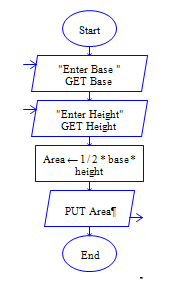# Area of Right angled triangle in Python

Python program to get length and width of right angled trianlgle and find area of right angled triangle

Sample Input 1:

4 5

Sample Output 1:

10

#### Flow Chart Design#### Program or Solution

``` l=int(input("Enter the length:")) w=int(input("Enter the width:")) area=(l*w)/2 print("Area: {}".format(area)) ```

#### Program Explanation

get length and width of a right-angled triangle using input() method calculate area = length * width